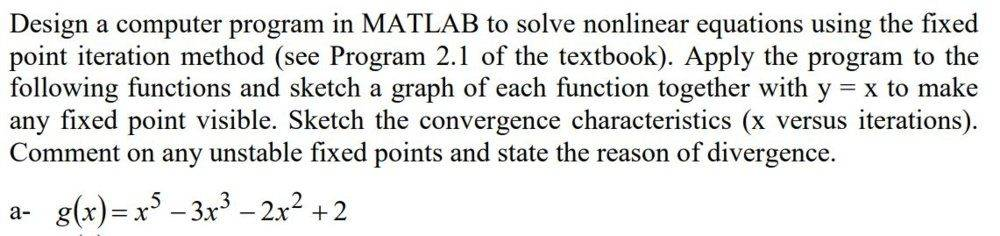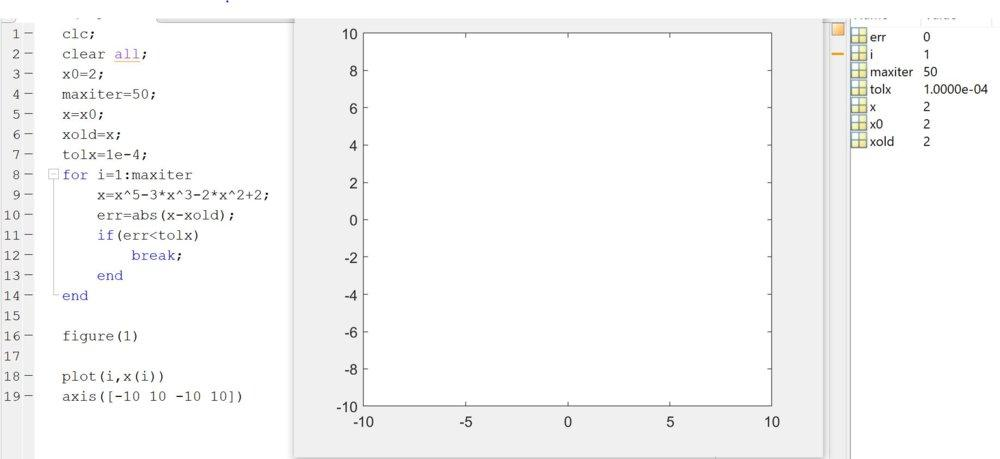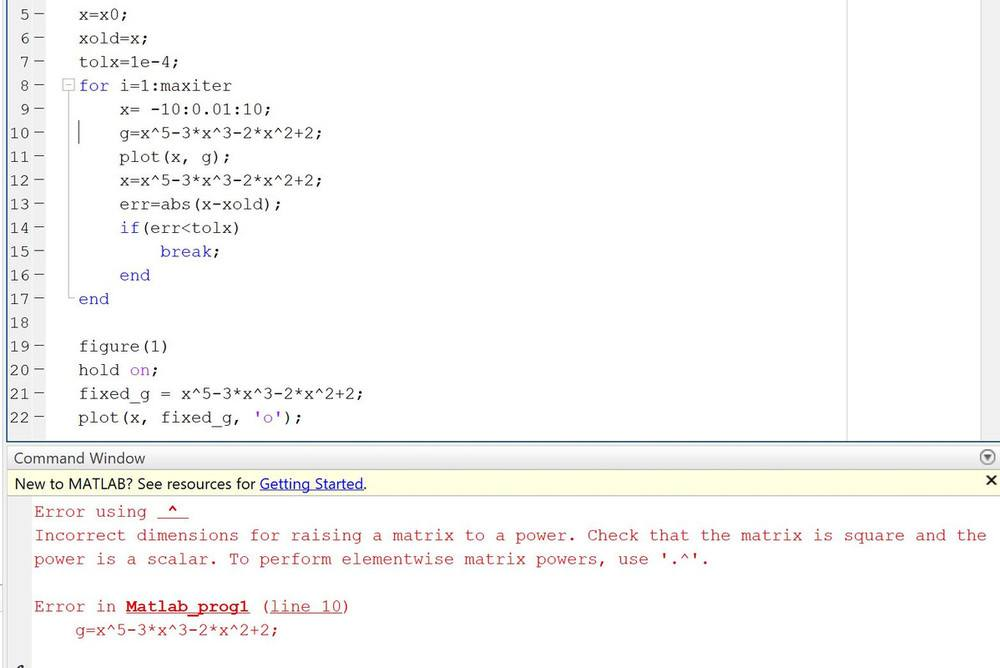# Solve a nonlinear equation using fixed-point iteration in MATLAB

• Engineering
Fatima Hasan
Homework Statement:
The statement is attached below.
Relevant Equations:
-
My attempt is attached below.
When I tried to solve it , nothing comes up. However, there are no errors !Jaime_mc2
You are plotting just one point at the end, with coordinates (maxiter + 1, x(maxiter + 1)).

If you want to plot ##g(x)##, you should do something like this (for example before calculating the fixed-point):
Matlab:
x= -10:0.01:10;
g = x^5-3*x^3-2*x^2+2;
plot(x, g);

After this, you should calculate the fixed-point. If you call it ##x## in your code, you can do the following after the fixed-point iteration:
Matlab:
hold on;
fixed_g = x^5-3*x^3-2*x^2+2;
plot(x, fixed_g, 'o');

Fatima Hasan
g = x^5-3*x^3-2*x^2+2;
I got an error .Fatima Hasan
g = x^5-3*x^3-2*x^2+2;
I got an error .